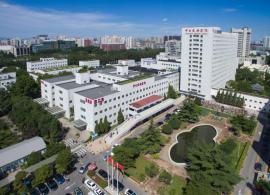# 医院主页• 骨科门诊
• 消化内科门诊
• 呼吸病门诊
• 中医风湿病科门诊
• 儿科门诊
• 肛肠科门诊
• 胸外科门诊
• 神经外科门诊
• 普通外科门诊
• 脊柱外科门诊
• 整形外科门诊
• 呼吸病门诊(特需)
• 耳鼻喉科门诊
• 疼痛门诊
• 神经内科门诊
• 肾内科门诊
• 心内科门诊
• 骨关节外科门诊
• 皮肤病与性病科门诊
• 美容外科门诊
• 眼科门诊
• 中医肺病一部门诊
• 男科门诊
• 泌尿外科
• 妇科门诊
• 男科门诊(特需)
• 中西医结合肿瘤内科门诊
• 消化内科门诊(特需)
• 口腔科门诊
• 肝胆胰疾病(肝胆外科)
• 普通内科门诊
• 普通外科门诊(特需)
• 神经科门诊(特需)
• 中西医心内科
• 放射治疗科门诊
• 中医针灸科门诊
• 血液科门诊
• 中医脾胃病科门诊
• 急诊科
• 骨科门诊(特需)
• 头颈肿瘤(耳鼻喉)
• 肾病科门诊(特需)
• 临床戒烟中心戒烟门诊
• 神经外科门诊(特需)
• 乳腺甲状腺外科(特需)
• 风湿免疫科门诊
• 内分泌科门诊
• 干部医疗科
• 口腔医学中心
• 心脏科门诊(特需)
• 普通内科（心内科）
• 心脏内科
• 疼痛门诊(特需)
• 内窥镜室
• 妇产科
• 普通外科一部
• 中医糖尿病科门诊(特需)
• 中医肺病二科门诊
• 风湿免疫科门诊(特需)
• 内分泌科门诊(特需)
• 内分泌科中医门诊
• 心脏·血管外科门诊
• 口腔正畸
• 感染科门诊
• 麻醉科
• 乳腺甲状腺专病(普二)
• 美容牙科门诊
• 乳腺病门诊(乳甲科)
• 心理咨询门诊
• 中医风湿病科(特需)
• 肛肠科
• 口腔种植(口腔科)
• 美容牙科(种植)
• 神经、癫痫门诊
• 整形外科门诊(特需)
• 普外四病区门诊
• 皮肤性病科
• 放射诊断科门诊
• 皮肤病与性病科门诊(特需)
• 疼痛科
• 脊柱外科门诊(特需)
• 骨关节外科门诊(特需)
• 胸外科
• 头痛门诊
• 呼吸与危重症医学科
• 康复医学科门诊
• 老年医学科门诊
• 超声诊断科门诊
• 中医内科门诊
• 中医外科门诊
• 病理科门诊
• 中医按摩科门诊
• 中医妇科门诊
• 口腔修复(镶牙)
• 产科门诊
• 精准医学中心门诊
• 预防保健科门诊
• 介入超声微创治疗门诊
• 特需联合门诊
• 营养科门诊
• 口腔牙体牙髓
• 核医学科门诊
• 乳腺癌
• 美容皮肤科门诊
• 骨创伤科门诊
• 电化疗科门诊
• 美容牙科(正畸)
• 胃肠胰腺神经内分泌肿瘤
• 中西医心内科门诊(特需)
• 中西医肿瘤内科门诊(特需)
• 结直肠癌多学科联合门诊
• 中医肺病一部门诊(特需)
• 淋巴瘤门诊
• 美容牙科(全科)
• 宫颈病变、HPV感染专病
• 中医内科门诊(特需)
• 儿童口腔
• 口腔颌面外科门诊
• 儿童生长发育专病门诊
• 骨坏死中西医结合诊疗
• 肾上腺病多学科联合门诊
• 牙齿美容冷光漂白
• 神经眼科相关疾病
• 院士工作室预约
• 胆道门诊
• 银屑病(皮肤科)
• 肝功能异常
• 感染科门诊(特需)
• 激光美容整形专病门诊
• 美容牙科(修复)
• 呼吸病门诊(院士)
• 糖尿病与慢性气道联合门诊
• 中西医心衰
• 新早产儿脑损伤
• 肠道门诊
• 慢性心衰
• 心功能不全中西医联合门诊
• 血液透析门诊
• 血栓门诊(呼吸二)
• 肺部结节与肺癌(呼吸)
• 运动障碍神经遗传精准医学
• 耳聋耳鸣专病(耳鼻喉)
• 中医肺病二部门诊(特需)
• 口腔牙周
• 帕金森病多学科联合门诊
• 甲旁亢手术联合门诊
• 双心门诊（中西医心内科
• 神内放射联合门诊
• 神经眼科联合门诊
• 美容牙科(牙体)
• 胃食管反流病(普二)
• 日间手术[胆囊阑尾](普四)
• 中医睡眠障碍(老年科)
• 神内康复国疗联合门诊
• 消化道肿瘤联合门诊(特需)
• 川崎病精准医学门诊
• 肺结节肺癌全科门诊(放肿)
• 神内内分泌联合门诊
• 男科
• 口腔夜诊
• 神经视觉障碍
• 贲门失弛缓间质瘤门诊
• 胰胆疾病(消化)
• 腰腿痛微创治疗门诊(脊柱)
• 瘢痕专病(皮肤科)
• 美容牙科(牙周)
• 内分泌泌外血管外联合门诊
• 男性更年期健康中医门诊
• 胰腺疾病(联合门诊)
• 肝病、消化不良(中医消化)
• 脑血管病影像联合门诊
• 肿瘤微创联合门诊
• 盆腔炎专病(中医妇科)
• 糖尿病周围神经痛联合门诊
• 糖肾心脑联合门诊
• 咳嗽(中医呼吸科)
• 美容牙科(口腔外科)
• 预防保健科门诊(特需)
• 运动心肺康复门诊(康复)
• 月经紊乱及不孕联合门诊
• 癌痛门诊
• 呼吸困难门诊(呼吸)
• 矮小症的精准治疗
• 美容牙科(儿科)
• 呼吸困难与间质性肺病
• 呼吸感染专病(呼吸)
• 肺癌多学科联合门诊
• 呼吸心内联合门诊
• 运动障碍神经遗传联合门诊
• 内分泌呼吸老年联合门诊
• 血脂异常门诊
• 下尿路功能障碍(泌外)
• 疑难呼吸困难联合门诊
• 心功能不全中西医联合
• 血栓性疾病联合门诊